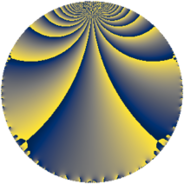# Properties

 Label 38.3.fLevel $38$ Weight $3$ Character orbit 38.f Rep. character $\chi_{38}(3,\cdot)$ Character field $\Q(\zeta_{18})$ Dimension $24$ Newform subspaces $1$ Sturm bound $15$ Trace bound $0$

# Related objects

## Defining parameters

 Level: $$N$$ $$=$$ $$38 = 2 \cdot 19$$ Weight: $$k$$ $$=$$ $$3$$ Character orbit: $$[\chi]$$ $$=$$ 38.f (of order $$18$$ and degree $$6$$) Character conductor: $$\operatorname{cond}(\chi)$$ $$=$$ $$19$$ Character field: $$\Q(\zeta_{18})$$ Newform subspaces: $$1$$ Sturm bound: $$15$$ Trace bound: $$0$$

## Dimensions

The following table gives the dimensions of various subspaces of $$M_{3}(38, [\chi])$$.

Total New Old
Modular forms 72 24 48
Cusp forms 48 24 24
Eisenstein series 24 0 24

## Trace form

 $$24q - 6q^{3} + 12q^{6} - 18q^{7} + 6q^{9} + O(q^{10})$$ $$24q - 6q^{3} + 12q^{6} - 18q^{7} + 6q^{9} + 30q^{11} - 36q^{12} - 90q^{13} - 48q^{14} - 114q^{15} + 18q^{17} - 12q^{19} + 24q^{20} + 90q^{21} + 84q^{22} + 120q^{23} - 24q^{24} + 252q^{25} + 48q^{26} + 126q^{27} + 72q^{28} - 210q^{29} - 108q^{31} - 132q^{33} - 24q^{34} - 66q^{35} - 12q^{36} + 84q^{38} + 120q^{39} + 54q^{41} + 72q^{42} + 90q^{43} - 48q^{44} - 144q^{45} - 360q^{46} - 246q^{47} - 48q^{48} + 54q^{49} - 432q^{50} - 342q^{51} + 36q^{52} - 174q^{53} - 42q^{55} - 12q^{57} + 48q^{58} + 228q^{59} + 132q^{60} + 12q^{61} + 204q^{62} + 174q^{63} + 96q^{64} + 630q^{65} + 696q^{66} + 72q^{67} - 48q^{68} + 702q^{69} + 528q^{70} + 432q^{71} + 96q^{72} - 144q^{74} + 72q^{76} - 144q^{77} - 708q^{78} - 246q^{79} - 642q^{81} - 384q^{82} - 126q^{83} - 540q^{84} - 684q^{85} - 12q^{86} - 324q^{87} - 12q^{89} - 336q^{90} + 372q^{91} - 132q^{92} - 168q^{93} - 570q^{95} + 72q^{97} + 384q^{98} - 204q^{99} + O(q^{100})$$

## Decomposition of $$S_{3}^{\mathrm{new}}(38, [\chi])$$ into newform subspaces

Label Dim. $$A$$ Field CM Traces $q$-expansion
$$a_2$$ $$a_3$$ $$a_5$$ $$a_7$$
38.3.f.a $$24$$ $$1.035$$ None $$0$$ $$-6$$ $$0$$ $$-18$$

## Decomposition of $$S_{3}^{\mathrm{old}}(38, [\chi])$$ into lower level spaces

$$S_{3}^{\mathrm{old}}(38, [\chi]) \cong$$ $$S_{3}^{\mathrm{new}}(19, [\chi])$$$$^{\oplus 2}$$